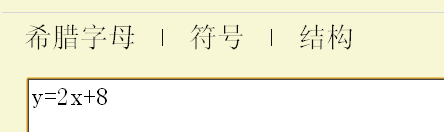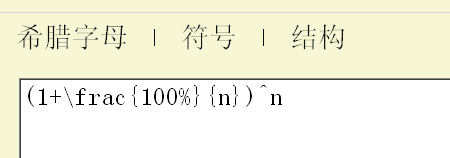# 数学公式生成器（完）

## 留言（31条）

$latex (1+\frac{100\%}{n})^n$

$latex \big(1+\frac{100\%}{n}\big)^n$

$latex \displaystyle (1+\frac{100\%}{n})^n$

$latex \displaystyle \Big(1+\frac{100\%}{n}\Big)^n$

http://www.math.union.edu/~dpvc/jsMath/

Mathjax

Wonderful.

@Denny：

To 阮一峰,

WolframAlpha早就支持普通表达式和latex，同时也可以作图解方程等等。
http://www.wolframalpha.com/input/?i=%281%2B%5Cfrac{100%25}{n}%29^n

http://www.qiujingyu.com/insert-math-formula-into-web-page.html

«-必填

«-必填，不公开

«-我信任你，不会填写广告链接